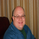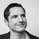# Automatic Daily Fibonacci v0.3 by JustUncleL

จำนวนเข้าชม 11361
This update is a correction on the way the Fib Zero line is calculated. Previously it was just based on the source of the previous day, which is Ok as long as the Fiblength was set to 2. The new version calculates the Fib Zero from the average of the specified source over the Fiblength, so if Fiblength is now something other than 2 the Fibonacci Zero and other support and resistant levels should calculate correctly.
```//@version=2
// Name: Automatic Daily Fibonacci v0.3
// By: JustUncleL
// Date: 14-Jul-2016
// Version: v0.3
//
// Description:
//   I was looking for a Indicator that would draw Fibonacci retracement levels
//   based on the previous days highest and lowest point automatically, then
//   update the levels if with current days high and low dynamically.
//   I was unable to find exactly what I was looking for so made this one.
//   The following code actually performs the task that I used to do
//   manually, this saves me set up time and avoids errors.
//   I achieved this by combining some of the ideas from two other very
//   similar indicators (thank you for code hope you don't mind):
//   - Auto Fib by TheYangGuizi
//   - [RS]Monthly Dynamic Range Levels (Fibonaci) V0 by RicardoSantos
//
// Modifications:
//   v0.3 (18-Sep-2016)
//   - Change the Fib zero to be the average of specified source over the "fiblength",
//     this caters better when fiblength is different from 2.
//   v0.2 (25-Jul-2016)
//   - Change Time frame type from "resolution" to "string", "resolution" was
//     restricting my time frame selections, I wanted to select 480(4H) and 720(8H)etc.
//     however, there is now no built-in resolution checking only valid ones can be
//     entered for axample: 60, 120, 240, 360, 480, 720, D, W, M, 2D, 2W, 3M...
//   - Change default zero from (hlc3) to (hl2), seems to give better results.
//   v0.1 (20-Jul-2016)
//   - Corrections and added optional source for centre line, it will
//     take the previous days position as centre line (hlc3), the original used
//     current days openning, but this could influenced by a spike.
//
study(title="Automatic Daily Fibonacci v0.3 by JustUncleL", shorttitle="AutoDailyFib v0.3 by JustUncleL", overlay=true, scale=scale.right)
//
// Get the time frame we are basing the Fibonacci retracements levels on.
// By default use Daily current day and previous day.
TimeFrame = input('1D',type=string,title="Timeframe For Fib Levels" )
fiblength=input(2,minval=1,title="Fibonacci Lookback Length")
zeroSrc = input(hl2,title="Fibonacci Centre Line Source")

//
// Find the highest and lowest 2 days(by default), including current.
// Start Fib retracement from average source over fiblength (default:hl2)
Z_000 = security(tickerid, TimeFrame, sum(zeroSrc,fiblength)/fiblength)
H_100 = security(tickerid, TimeFrame, highest(high,fiblength))
L_100 = security(tickerid, TimeFrame, lowest(low,fiblength))

// Now Range the Fib arround today's openning price.
H_RANGE = H_100-Z_000
L_RANGE = Z_000-L_100

// Calculate Fib Upper points from top down to zero
H_764 = H_100 - H_RANGE * 0.236
H_618 = H_100 - H_RANGE * 0.382
H_500 = H_100 - H_RANGE * 0.500
H_382 = H_100 - H_RANGE * 0.618
H_236 = H_100 - H_RANGE * 0.764

// Calculate Fib Lower points from bottom up to zero
L_764 = L_100 + L_RANGE * 0.236
L_618 = L_100 + L_RANGE * 0.382
L_500 = L_100 + L_RANGE * 0.500
L_382 = L_100 + L_RANGE * 0.618
L_236 = L_100 + L_RANGE * 0.764

// Plot all the upper and lower Fib lines dynamically
h100=plot( H_100, title="+1.000", color=black, transp=30, linewidth=2)
h764=plot( H_764, title="+0.764", color=yellow, transp=0)
h618=plot( H_618, title="+0.618", color=blue, transp=0)
h500=plot( H_500, title="+0.500", color=lime, transp=0)
h382=plot( H_382, title="+0.382", color=green, transp=0)
h236=plot( H_236, title="+0.236", color=red, transp=0)
zero=plot( Z_000, title="0.000",  color=black, transp=70, linewidth=2,style=cross,join=true)
l236=plot( L_236, title="-0.236", color=red, transp=0)
l382=plot( L_382, title="-0.382", color=green, transp=0)
l500=plot( L_500, title="-0.500", color=lime, transp=0)
l618=plot( L_618, title="-0.618", color=blue, transp=0)
l764=plot( L_764, title="-0.764", color=yellow, transp=0)
l100=plot( L_100, title="-1.000", color=black, transp=30, linewidth=2)

// Let's make it all look good fill in the gaps.
fill(h100,h764, color=maroon, transp=90)
fill(h764,h618, color=yellow, transp=90)
fill(h618,h500, color=blue, transp=90)
fill(h500,h382, color=lime, transp=90)
fill(h382,h236, color=green, transp=90)
fill(h236,zero, color=red, transp=90)
fill(zero,l236, color=red, transp=90)
fill(l382,l236, color=green, transp=90)
fill(l500,l382, color=lime, transp=90)
fill(l618,l500, color=blue, transp=90)
fill(l764,l618, color=yellow, transp=90)
fill(l764,l100, color=maroon, transp=90)
//
//EOF.```
JustUncleL

## ความคิดเห็นjianhua85
@jianhua85, It could possibly be performed, but it will not be related to the intention of this script though.
ตอบกลับpratheepnz